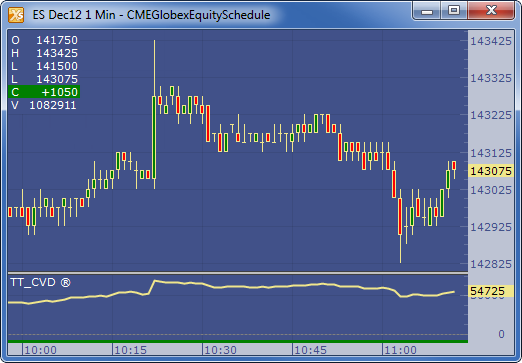← Back to X_TRADER® Help Library

### X_STUDY® Documentation

#### TT Cumulative Vol Delta (TT CVD)

##### Description

The Cumulative Volume Delta (TT CVD Study) displays a running total of net transactions as calculated by Volume Delta. Transactions occurring on the Ask are considered Buying Pressure and are added to the total, and those occurring on the Bid are considered Selling Pressure and are subtracted from the cumulative total.

##### Formula

TT CVD = Cumulative ( Vol ∆ )

Vol ∆ = Difference between Bid Volume and Ask volume over specified interval

Bid Volume = - ( Accumulated Bid Volume )